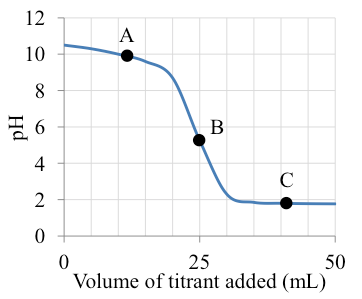# Problem: The titration curve shown below represents a 25 mL aqueous solution that is titrated with another solution at the same molarity. Which of the following statement is TRUE regarding the titration?A. The curve could represent the titration of NaOH with HCl (adding HCl to NaOH)B. The equivalence point is at pH 7C. Point A is in the buffer regionD. At point C, there is excess base presentE. The curve could represent the titration of H2SO4 with NaOH (adding NaOH to H2SO4)

###### FREE Expert Solution
86% (255 ratings)
###### Problem Details

The titration curve shown below represents a 25 mL aqueous solution that is titrated with another solution at the same molarity. Which of the following statement is TRUE regarding the titration?

A. The curve could represent the titration of NaOH with HCl (adding HCl to NaOH)

B. The equivalence point is at pH 7

C. Point A is in the buffer region

D. At point C, there is excess base present

E. The curve could represent the titration of H2SO4 with NaOH (adding NaOH to H2SO4)What scientific concept do you need to know in order to solve this problem?

Our tutors have indicated that to solve this problem you will need to apply the Acid and Base Titration Curves concept. You can view video lessons to learn Acid and Base Titration Curves. Or if you need more Acid and Base Titration Curves practice, you can also practice Acid and Base Titration Curves practice problems.

What is the difficulty of this problem?

Our tutors rated the difficulty ofThe titration curve shown below represents a 25 mL aqueous s...as medium difficulty.

How long does this problem take to solve?

Our expert Chemistry tutor, Jules took 3 minutes and 34 seconds to solve this problem. You can follow their steps in the video explanation above.

What professor is this problem relevant for?

Based on our data, we think this problem is relevant for Professor Ratliff's class at USF.